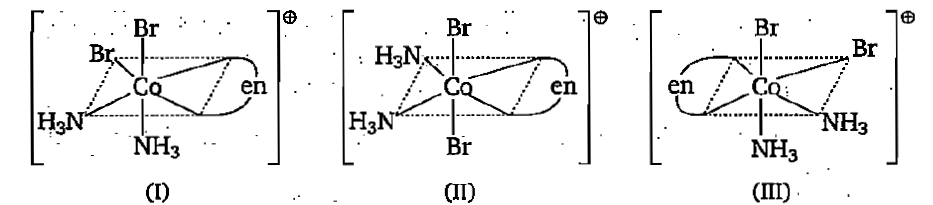Three arrangements are shown for the complex $\left[{\mathrm{CoBr}}_{2}\left({\mathrm{NH}}_{3}{\right)}_{2}\left(\mathrm{en}\right){\right]}^{\oplus }$. Which one is wrong statement?(a) I and II are geometrical isomers

(b) II and III are optically active isomers

(c) I and III are optically active isomers

(d) II and III are geometrical isomers

Concept Questions :-

VBT & CFT, Limitation /Hybridisation
High Yielding Test Series + Question Bank - NEET 2020

Difficulty Level:

A complex with the composition $\left[{\mathrm{MA}}_{3}\mathrm{B}{\right]}^{\mathrm{n}±}$ is found to have no geometrical isomers. The possible structures (s) of the complex is (Where A and B are monodentate ligands)

(a) Tetrahedral                    (b) Square planar

(c) Both (a) and (b)             (d) Cannot be predicted

Concept Questions :-

VBT & CFT, Limitation /Hybridisation
High Yielding Test Series + Question Bank - NEET 2020

Difficulty Level:

Complexes formed in the following methods are:

(I) Mond's process for purification of nickel

(II) Removal of unreacted AgBr from photographic plate

(III) Removal of lead poisoning from the body

Options are as follows:

Concept Questions :-

Coordination comp,Introduction and Classification / Nomenclature
High Yielding Test Series + Question Bank - NEET 2020

Difficulty Level:

The correct for the name for the complex ion $\left[\mathrm{CoCl}\left(\mathrm{ONO}\right)\left(\mathrm{en}{\right)}_{2}{\right]}^{+}$ is:

(a) chlorobis(ethylenediamine)nitrito-O-cobaltate(III) ion

(b) chlorodiethyldiaminenitrito-O-cobalt(III) ion

(c) chloronitrito-O-diethyldiamine cobaltate (III) ion

(d) chlorobis (ethylenediamine)nitrito-O-cobalt (III) ion

High Yielding Test Series + Question Bank - NEET 2020

Difficulty Level:

IUPAC name of ${\mathrm{H}}_{2}\left[{\mathrm{PtCl}}_{6}\right]$ is:

(a) hydrogen hexachloroplatinate (IV)

(b) dihydrogen hexachloroplatinate (IV)

(c) hydrogen hexachloroplatinic (IV) acid

(d) hexachloroplatinic (IV) acid

Concept Questions :-

Coordination comp,Introduction and Classification / Nomenclature
High Yielding Test Series + Question Bank - NEET 2020

Difficulty Level:

Which of the following ligand does not as $\mathrm{\pi }$-acid ligand?

(a) ${\mathrm{N}}_{2}$           (b) CO              (c) ${\mathrm{C}}_{2}{\mathrm{H}}_{4}$                 (d) ${\mathrm{O}}_{2}^{2-}$

Concept Questions :-

Ligands
High Yielding Test Series + Question Bank - NEET 2020

Difficulty Level:

$\left[\mathrm{Mn}\left(\mathrm{CO}{\right)}_{4}\mathrm{NO}\right]$ is diamagnetic because:

(a) Mn metal is diamagnetic in free state

(b) Mn is in +1 oxidation state in this complex

(c) NO is present as positive ligand

(d) All of the above

Concept Questions :-

VBT & CFT, Limitation /Hybridisation
High Yielding Test Series + Question Bank - NEET 2020

Difficulty Level:

Choose the correct option regarding the following complex compound which follows (F) and does not follow (NF) the Sidgwick EAN rule:

(I) $\left[\left({\mathrm{Ph}}_{3}\mathrm{P}{\right)}_{2}{\mathrm{PdCl}}_{2}{\mathrm{PdCl}}_{2}\right]$                     (II) $\left[\mathrm{NiBrCl}\left(\mathrm{en}\right)\right]$

(III) ${\mathrm{Na}}_{4}\left[\mathrm{Fe}\left(\mathrm{CN}{\right)}_{5}\mathrm{NOS}\right]$                       (IV) $\mathrm{Cr}\left(\mathrm{CO}{\right)}_{3}\left(\mathrm{NO}{\right)}_{2}$

(I)    (II)    (III)    (IV)                       (I)    (II)    (III)     (IV)

(a)    NF   NF      NF       NF                 (b)    F       F        NF        F

(c)     NF   NF      F          F                  (d)   NF      NF      F          NF

Concept Questions :-

E.A.N
High Yielding Test Series + Question Bank - NEET 2020

Difficulty Level:

If CO ligands are substituted by NO in respective neutral carbonyl compounds then which of the following will not be correct formula ?

(a) $\mathrm{Cr}\left(\mathrm{CO}{\right)}_{3}\left(\mathrm{NO}{\right)}_{2}$                       (b) $\mathrm{Fe}\left(\mathrm{CO}{\right)}_{2}\left(\mathrm{NO}{\right)}_{2}$

(c) $\mathrm{Cr}\left(\mathrm{NO}{\right)}_{4}$                                (d) $\mathrm{Ni}\left(\mathrm{CO}{\right)}_{2}\left(\mathrm{NO}{\right)}_{2}$

Concept Questions :-

Ligands
High Yielding Test Series + Question Bank - NEET 2020

Difficulty Level:

Which of the following species can act as reducing agent ?

(a) $\left[\mathrm{Co}\left(\mathrm{CO}{\right)}_{4}{\right]}^{-}$      (b) $\mathrm{Mn}\left(\mathrm{CO}{\right)}_{6}$       (c) $\mathrm{Mn}\left(\mathrm{CO}{\right)}_{5}$       (d) $\mathrm{Cr}\left(\mathrm{CO}{\right)}_{6}$

Concept Questions :-

E.A.N